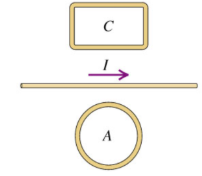# Problem: Two closed loops A and C are close to a long wire carrying a current IWhile I is decreasing, what is the direction of the net force that the wire exerts on loop A?

###### FREE Expert Solution

If the current is decreasing, there will be induced current in loop A, which is in a clockwise direction.

Therefore, loop A gets an attractive force.

81% (306 ratings)###### Problem Details

Two closed loops A and C are close to a long wire carrying a current IWhile I is decreasing, what is the direction of the net force that the wire exerts on loop A?